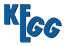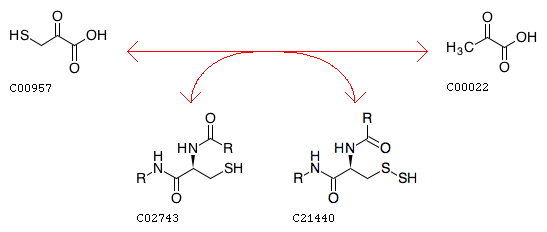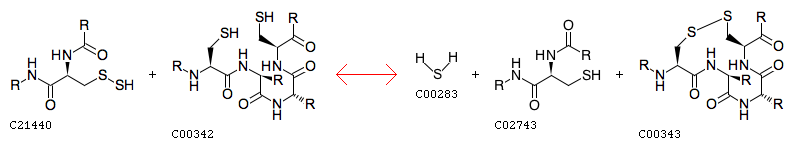REACTION: R12689
Entry
 ```R12689                      Reaction                                ```
Definition
Mercaptopyruvate + [Protein]-L-cysteine <=> Pyruvate + [Protein]-S-sulfanyl-L-cysteine
EquationComment
the first step of two-step reaction (see R03105, R12689+R12690)
Reaction class
 C00022_C00957
EnzymeREACTION: R12690
Entry
 ```R12690                      Reaction                                ```
Definition
[Protein]-S-sulfanyl-L-cysteine + Thioredoxin <=> Hydrogen sulfide + [Protein]-L-cysteine + Thioredoxin disulfide
EquationComment
the second step of two-step reaction (see R03105, R12689+R12690)
Enzyme